Examples

Chapter 3 Class 10 Pair of Linear Equations in Two Variables
Serial order wise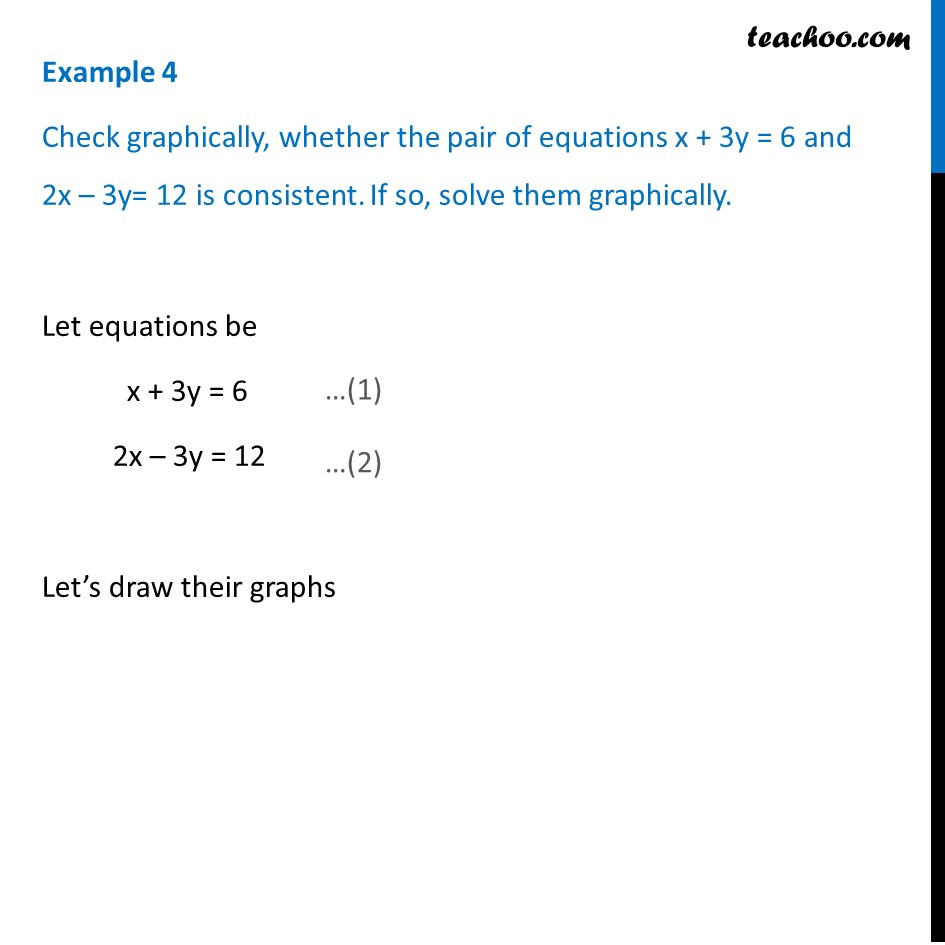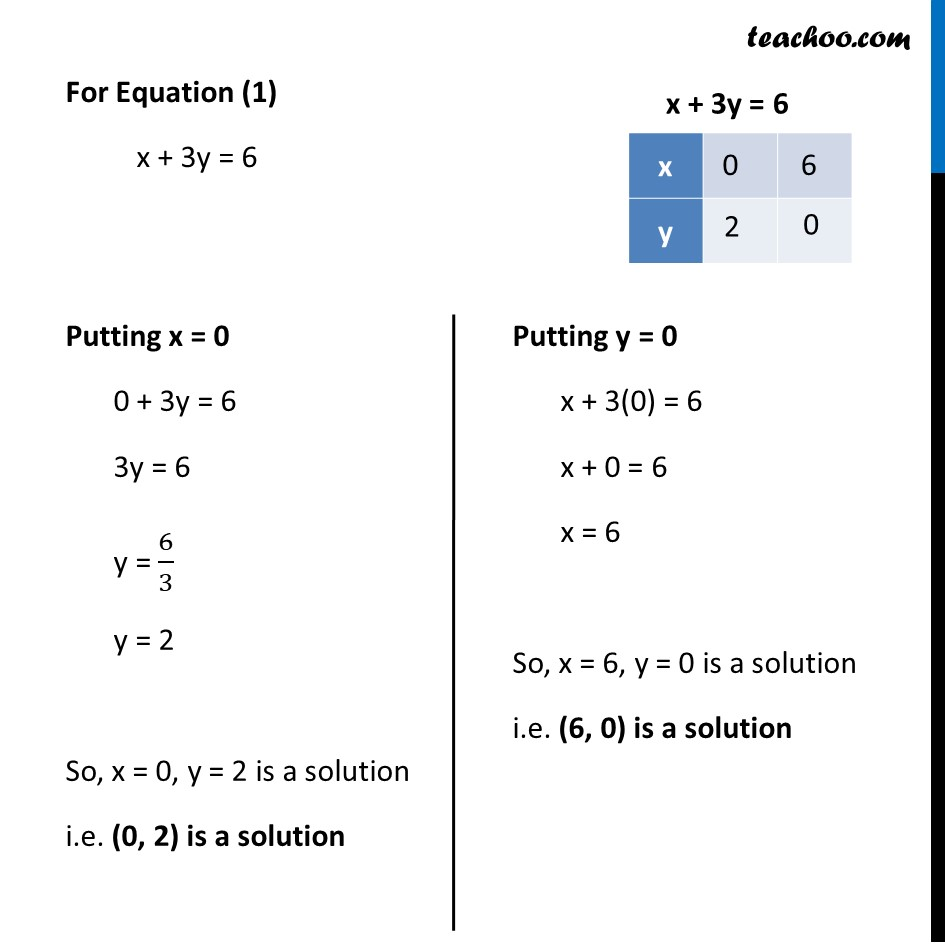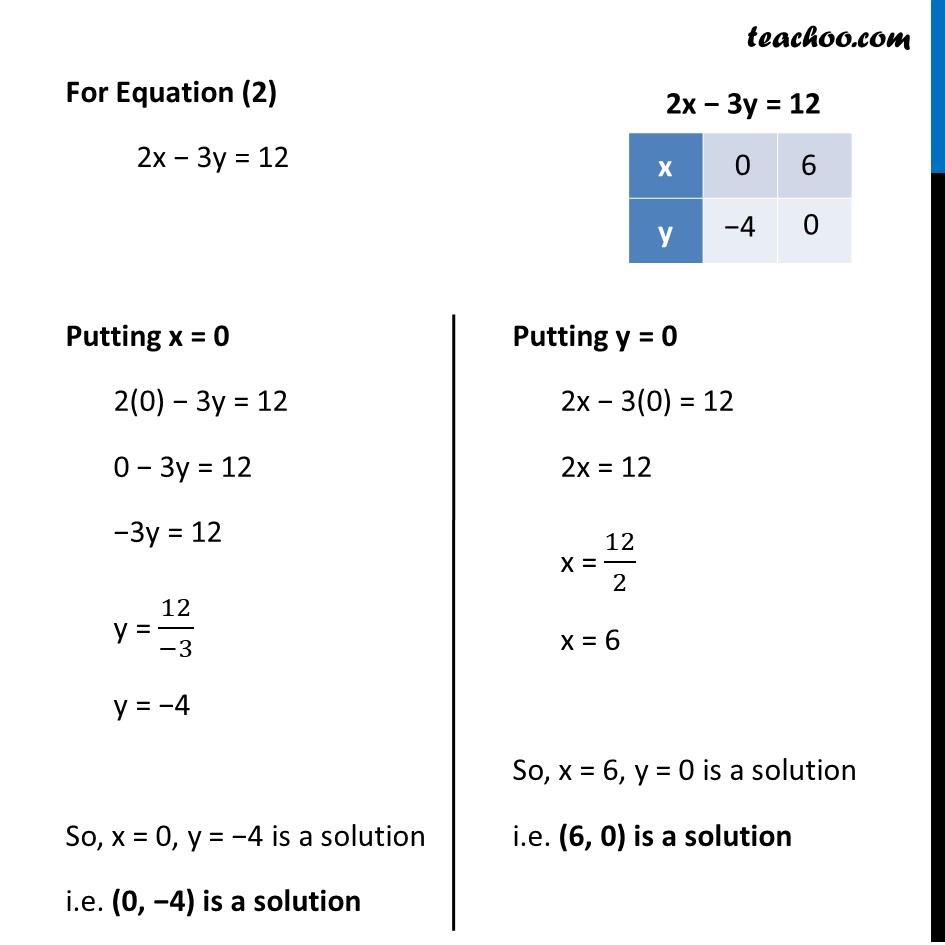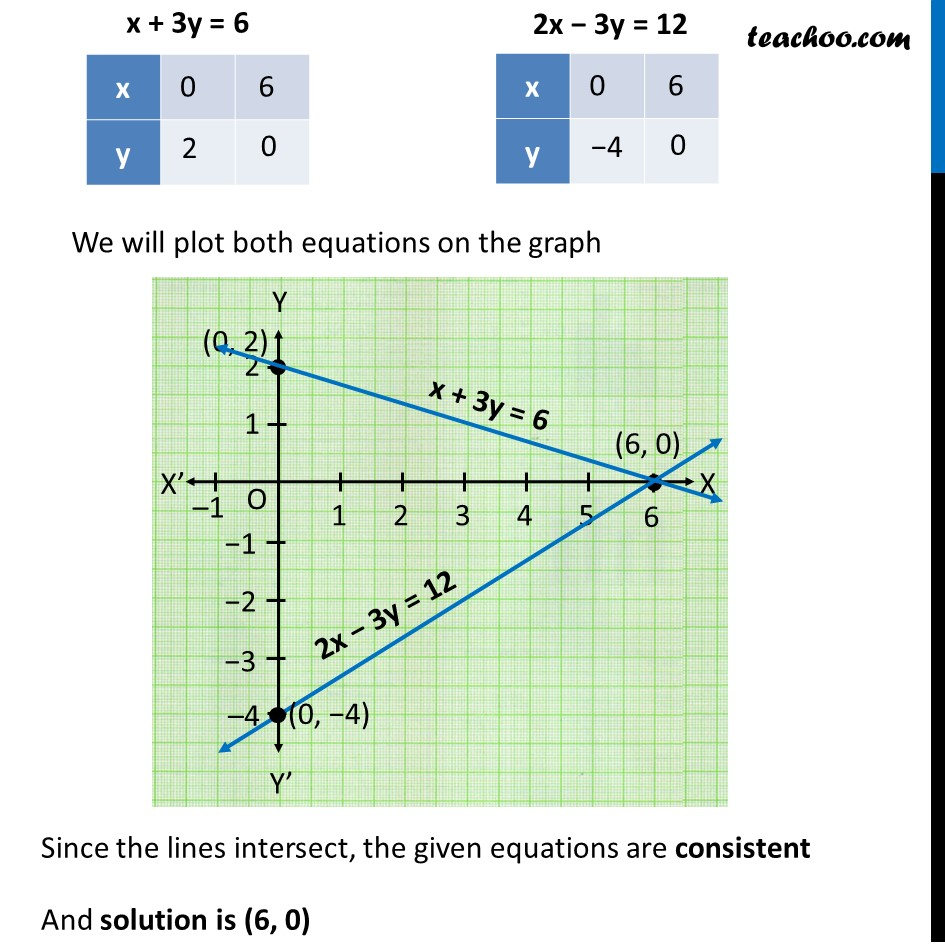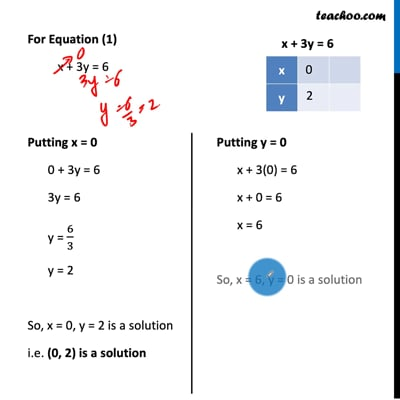This video is only available for Teachoo black users

Solve all your doubts with Teachoo Black (new monthly pack available now!)

### Transcript

Example 4 Check graphically, whether the pair of equations x + 3y = 6 and 2x – 3y= 12 is consistent. If so, solve them graphically. Let equations be x + 3y = 6 2x – 3y = 12 Let’s draw their graphs For Equation (1) x + 3y = 6 Putting x = 0 0 + 3y = 6 3y = 6 y = 6/3 y = 2 So, x = 0, y = 2 is a solution i.e. (0, 2) is a solution Putting y = 0 x + 3(0) = 6 x + 0 = 6 x = 6 So, x = 6, y = 0 is a solution i.e. (6, 0) is a solution For Equation (2) 2x − 3y = 12 Putting x = 0 2(0) − 3y = 12 0 − 3y = 12 −3y = 12 y = 12/(−3) y = −4 So, x = 0, y = −4 is a solution i.e. (0, −4) is a solution Putting y = 0 2x − 3(0) = 12 2x = 12 x = 12/2 x = 6 So, x = 6, y = 0 is a solution i.e. (6, 0) is a solution We will plot both equations on the graph Since the lines intersect, the given equations are consistent And solution is (6, 0)